# Line

How many parts of line divide 5 (different) points that lie on it?

n =  6

### Step-by-step explanation:

$n=5+1=6$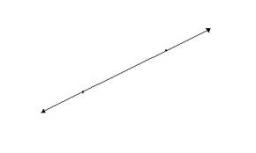Did you find an error or inaccuracy? Feel free to write us. Thank you!## Related math problems and questions:

• Plane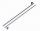On how many parts divide plane 6 parallels?
• Draw it!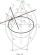Draw two lines c, d that c || d. On line c, mark the points A, B. By point A, a lead perpendicular line to c. By point B, lead perpendicular line to c.
• DivideDivide substance 110 cm long to two parts so that it first part is 10 cm longer than the second part and one part will be 10 times longer than the second portion. How long the parts will be?
• Three points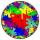Mark three points E, F and G in the plane not lie on one line. a) Draw a line segment FG b) Construct halfline (ray) EG c) Draw a line EF
• Pizza master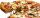Master says that he can splits pizza to 16 parts by five equals straight cuts. Is it possible?
• Dividing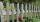Divide the three-line segment 13 cm, 26 cm, and 19.5 cm long for parts so that the individual pieces were equally long and longest. How long will the individual parts, and how many will it?
• Camping tripA group of friends on a camping trip has 3 2/5 lb of trail mix. They divide it up evenly among themselves. Each person gets 1/5 lb of the trail mix. How many friends are on the camping trip?
• Class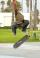The class has 18 students. Everyone knows inline skating or skateboarding. Inline skating can ride 11 students on a skateboard 10. How many ride on inline skates and on skateboard?
• LinesHow many lines can be draw with 8 points, if three points lie on one line and the other any three points do not lie on the same line?
• Civil protection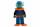Students on civil protection exercise went 5800 m long trip. How many kilometers is it approximately?
• Chocolate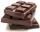How many times do we have to break a chocolate bar composed of 10 × 12 pieces to get the 120 parts?
• Straight lines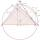Draw two lines c, d so that c || d. On line c mark points A, B, from point A start perpendicular to line c, from point B perpendicular to line c.
• Time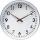16.2 days ..... how many hours is it?
• Expression 1What is 7+8-(5×2)+5-4+(6×(5-3)+6)-(8+10)-7+6?
• DozenWhat is the product of 26 and 5? Write the answer in an Arabic numeral. Add up the digits. How many of this is in a dozen? Divide #114 by this
• Points in planeThe plane is given 12 points, 5 of which is located on a straight line. How many different lines could by draw from this points?
• Points in space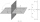There are n points, of which no three lie on one line and no four lies on one plane. How many planes can be guided by these points? How many planes are there if there are five times more than the given points?